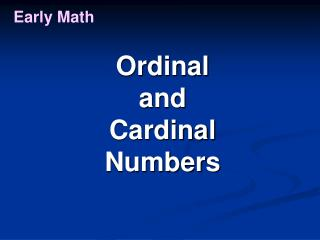DownloadDownload PresentationEarly Math

# Early Math

Télécharger la présentation## Early Math

- - - - - - - - - - - - - - - - - - - - - - - - - - - E N D - - - - - - - - - - - - - - - - - - - - - - - - - - -
##### Presentation Transcript

1. Early Math Ordinal and Cardinal Numbers

2. Early Math Ordinal Numbers Move one

3. Early Math Ordinal Numbers 1st Ring FIRST

4. Early Math Ordinal Numbers Move one more

5. Early Math Ordinal Numbers 2nd Ring SECOND

6. Early Math Ordinal Numbers Move one more

7. Early Math Ordinal Numbers 3rd Ring THIRD

8. Early Math Ordinal Numbers Move one more

9. Early Math Ordinal Numbers 4th Ring FOURTH

10. Early Math Ordinal Numbers Move one more

11. Early Math Ordinal Numbers 5th Ring FIFTH

12. Early Math Ordinal Numbers Move one more

13. Early Math Ordinal Numbers 6th Ring SIXTH

14. Early Math Ordinal Numbers Move one more

15. Early Math Ordinal Numbers 7th Ring SEVENTH

16. Early Math Ordinal Numbers Move one more

17. Early Math Ordinal Numbers 8th Ring EIGHTH

18. Early Math Ordinal Numbers Move one more

19. Early Math Ordinal Numbers 9th Ring NINETH

20. Early Math Ordinal Numbers Move one more

21. Early Math Ordinal Numbers 10th Ring TENTH

22. Early Math Don’t forget: Use MathLine along with other ordinal number exercises

23. Early Math Cardinal Numbers On MathLine Is the same as counting on MathLine